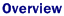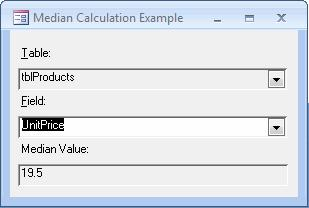# Finding the Median Value for Fields in Access 2007

This content is outdated and is no longer being maintained. It is provided as a courtesy for individuals who are still using these technologies. This page may contain URLs that were valid when originally published, but now link to sites or pages that no longer exist.

Summary: Learn how to calculate the median value in Microsoft Office Access 2007 fields by importing a custom module that contains a DMedian function. (7 printed pages)

Ken Getz, MCW Technologies, LLC

April 2009Although Microsoft Office Access 2007 provides the DAvg function to calculate a mean value, there is no equivalent function to calculate the median of a set of values. This article includes a VBA module that contains a custom function, DMedian, that calculates the median and emulates other domain functions like DSum, DMin, and DMax.Watch the Video Length: 06:19 | Size: 7.56 MB | Type: WMV file

Code It | Read It | Explore It

## Code It

Office Access 2007 does not provide a built-in function that calculates the median of a set of values, although if it did, the function might work something like the built-in DSum and DMax functions. This article demonstrates a median function, written in Visual Basic for Applications (VBA), that you can use in your own applications.

To try the function, first load the frmMedian form from the sample database, FindMedianValue.accdb. Choose a table and a field in that table by using the combo boxes on the form. After you choose a field, frmMedian uses the DMedian function to calculate the median value, and then displays that value in a text box. The form displays an error message if you choose a field with a nonnumeric data type, and displays the string "(Null)" if the median value is Null.

Figure 1. Sample form, frmMedian, displays the median UnitPrice valueFollow these steps to use the DMedian function in your own applications:

1. Import the basMedian module from FindMedianValue.accdb into your database.

2. Use the following table to determine what syntax to use to call the DMedian function. (It is similar to the built-in DAvg function.)

Table 1. DMedian Calling Syntax

 Parameter Description Example Field Name of field for which to calculate median "UnitPrice" Domain Name of a table or query "Products" Criteria Optional WHERE clause to limit the rows considered "CategoryID = 1"
3. Make sure that each parameter is delimited with quotes. The third parameter is optional. For example, you might enter the following statement in the Immediate window. The function call should return 18, assuming that you use the data in the sample database, FindMedianValue.accdb.

``````? DMedian("UnitPrice", "tblProducts", "SupplierID = 1")
``````Important

This example uses the DAO type library, and you’ll need to include the reference to the most current version of DAO in your own applications to take advantage of this code. Use the References command in the Tools menu to add the necessary reference, if you want to use this code in your own database.

The DMedian function in basMedian in FindMedianValue.accdb looks and acts similar to the built-in DAvg domain function. The algorithm that calculates the median, however, is more complicated, and uses the following steps:

1. Sort the dataset on the specified field.

2. Find the middle row of the dataset and return the value of the field. If there are an odd number of rows, this will be the value in a single row. If there are an even number of rows, there is no middle row, so the function finds the mean of the values in the two rows that straddle the middle. You could modify the function to pick an existing value instead.

After it declares a few variables, the DMedian function creates a recordset that is based on the three parameters that are passed to it (strField, strDomain, and varCriteria), as shown in the following complete source code for the function.

``````Public Function DMedian( _
ByVal strField As String, ByVal strDomain As String, _
Optional ByVal strCriteria As String) As Variant

' Purpose:
'     To calculate the median value
'     for a field in a table or query.
' In:
'     strField: the field.
'     strDomain: the table or query.
'     strCriteria: an optional WHERE clause to
'                  apply to the table or query.
' Out:
'     Return value: the median, if successful;
'                   Otherwise, an Error value.

Dim db As DAO.Database
Dim rstDomain As DAO.Recordset
Dim strSQL As String
Dim varMedian As Variant
Dim intFieldType As Integer
Dim intRecords As Integer

Const errAppTypeError = 3169

On Error GoTo HandleErr

Set db = CurrentDb()

' Initialize return value.
varMedian = Null

' Build SQL string for recordset.
strSQL = "SELECT " & strField & " FROM " & strDomain

' Only use a WHERE clause if one is passed in.
If Len(strCriteria) > 0 Then
strSQL = strSQL & " WHERE " & strCriteria
End If

strSQL = strSQL & " ORDER BY " & strField

Set rstDomain = db.OpenRecordset(strSQL, dbOpenSnapshot)

' Check the data type of the median field.
intFieldType = rstDomain.Fields(strField).Type
Select Case intFieldType
Case dbByte, dbInteger, dbLong, _
dbCurrency, dbSingle, dbDouble, dbDate
' Numeric field.
If Not rstDomain.EOF Then
rstDomain.MoveLast
intRecords = rstDomain.RecordCount
' Start from the first record.
rstDomain.MoveFirst

If (intRecords Mod 2) = 0 Then
' Even number of records.
' No middle record, so move to the
' record right before the middle.
rstDomain.Move ((intRecords \ 2) - 1)
varMedian = rstDomain.Fields(strField)
' Now move to the next record, the
' one right after the middle.
rstDomain.MoveNext
' And average the two values.
varMedian = _
(varMedian + rstDomain.Fields(strField)) / 2
' Make sure you return a date, even when
' averaging two dates.
If intFieldType = dbDate And Not IsNull(varMedian) Then
varMedian = CDate(varMedian)
End If
Else
' Odd number or records.
' Move to the middle record and return its value.
rstDomain.Move ((intRecords \ 2))
varMedian = rstDomain.Fields(strField)
End If
Else
' No records; return Null.
varMedian = Null
End If
Case Else
' Non-numeric field; so raise an app error.
Err.Raise errAppTypeError
End Select

DMedian = varMedian

ExitHere:
On Error Resume Next
rstDomain.Close
Set rstDomain = Nothing
Exit Function

HandleErr:
' Return an error value.
DMedian = CVErr(Err.Number)
Resume ExitHere
End Function
``````

Building the SQL string that defines the recordset is straightforward except for the construction of the optional WHERE clause. Because strCriteria is defined as an optional parameter (by using the Optional keyword), DMedian checks whether a value was passed by determining whether the string length is greater than zero.

``````' Build SQL string for recordset.
strSQL = "SELECT " & strField & " FROM " & strDomain

' Only use a WHERE clause if one is passed in.
If Len(strCriteria) > 0 Then
strSQL = strSQL & " WHERE " & strCriteria
End If

strSQL = strSQL & " ORDER BY " & strField
``````

Once DMedian builds the SQL string, it creates a recordset based on that SQL string.

``````Set rstDomain = db.OpenRecordset(strSQL, dbOpenSnapshot)
``````

Next, DMedian checks the data type of the field; it will calculate the median only for numeric and date/time fields. If you pass any other data type to DMedian, the function forces an error by calling the Err.Raise method and then uses the special CVErr function in its error handler to send the error state back to the calling procedure.

``````    ' Check the data type of the median field.
intFieldType = rstDomain.Fields(strField).Type
Select Case intFieldType
Case dbByte, dbInteger, dbLong, dbCurrency, _
dbSingle, dbDouble, dbDate
' Code removed here...
Case Else
' Non-numeric field; so raise an app error.
Err.Raise errAppTypeError
End Select

' Code removed here...

ExitHere:
On Error Resume Next
rstDomain.Close
Set rstDomain = Nothing
Exit Function

HandleErr:
' Return an error value.
DMedian = CVErr(Err.Number)
Resume ExitHere
End Function
``````

If the field is numeric, the DMedian function checks to see whether there are any rows in the recordset by using the following If...Then statement, and then returns Null if there are no rows.

``````' Numeric field.
If Not rstDomain.EOF Then
' Code removed here...
Else
' No records; return Null.
varMedian = Null
End If
``````

If there are rows, the function moves to the end of the recordset to get a count of the total number of records. This is necessary because the RecordCount property returns only the number of rows that have already been visited. The following is the code.

``````rstDomain.MoveLast
intRecords = rstDomain.RecordCount
``````

If the number of records is even, DMedian moves to the record just before the middle by using the Move method, which can move an arbitrary number of records from the current record. The number of records to move forward is calculated by using the following formula.

``````intRecords \ 2 - 1
``````

This tells Access to divide the total number of records by 2 and then subtract 1 from the result (because you are starting from the first record). For example, if you are on the first of 500 records, you would move (500 \ 2 - 1) = (250 - 1) = 249 records forward, which would bring you to the 250th record. Once the function has moved the specified number of records, it is a simple matter to grab the value of the 250th and 251st records and divide the result by 2. The following code shows this part of the function.

``````' Start from the first record.
rstDomain.MoveFirst

If (intRecords Mod 2) = 0 Then
' Even number of records. No middle record, so move
' to the record right before the middle.
rstDomain.Move ((intRecords \ 2) - 1)
varMedian = rstDomain.Fields(strField)
' Now move to the next record, the one right after
' the middle.
rstDomain.MoveNext
' Average the two values.
varMedian = (varMedian + rstDomain.Fields(strField)) / 2
``````

Because DMedian supports dates, the function uses the following code to make sure that it returns a date value when it takes the average of two dates.

``````' Make sure you return a date, even when
' averaging two dates.
If intFieldType = dbDate And Not IsNull(varMedian) Then
varMedian = CDate(varMedian)
End If
``````

The code for an odd number of rows is simpler, because the code merely needs to return the value of the middle row.

``````Else
' Odd number of records. Move to the middle record
' and return its value.
rstDomain.Move ((intRecords \ 2))
varMedian = rstDomain.Fields(strField)
End If
``````

Finally, acbDMedian returns the median value to the calling procedure.

``````acbDMedian = varMedian
``````